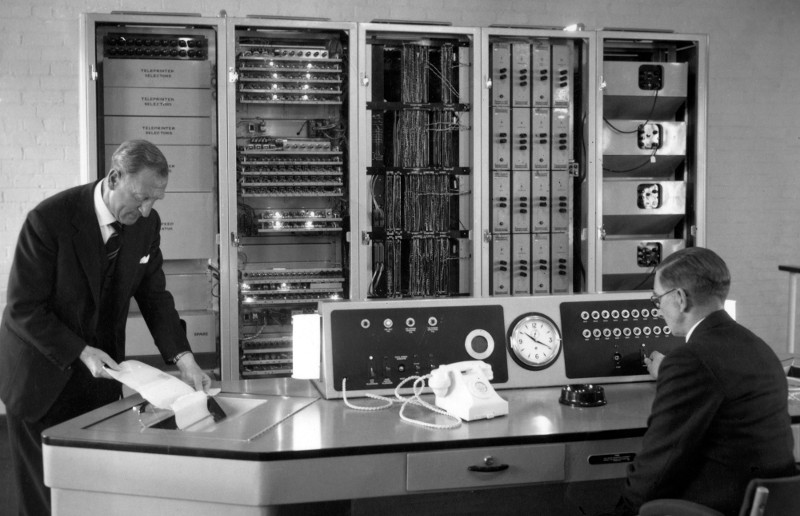#### What is Randomness?

There is no such thing as a random number. Rather, numbers in a sequence are considered "random" if they exhibit a high degree of variance from one another - and, crucially, if the sequence cannot be predicted. This is a problem for computers, which need random numbers for lots of things - and for the people who rely on them - because if you calculate a number, then it's always going to be predictable if you know what went into the calculation.

Most of the time, this isn't really a problem. Computer scientists have developed extremely sophisticated ways of creating pseudorandom numbers which are good enough for most applications, such as the Mersenne Twister. It's also possible to measure the electrical fluctuations in computer chips as a source of randomness. Random.org uses electrical noise in the atmosphere picked up by a radio.

The robots in A State of Sin use a variety of real-world sources that change, unpredictably, over time, such as the sound levels in the gallery, or different levels of gases in the atmosphere.

They are inspired by other "real" random number generators used in history, such as the Kleroterion of ancient Athens, which decided which citizens would participate in the original democracy. Lavarand was a true random number generator developed in the 1970s, which took photos of the wax floating in a lava lamp. The original ERNIE (Electronic Random Number Indicator Equipment - pictured above), which picked the winners in the Premium Bonds, used an array of flourescent tubes to create electrical noise. (ERNIE was designed at the Post Office Research Station by Tommy Flowers and Harry Fensom, and was based on the Colossus, the world's first digital computer.)

#### How the robots make random numbers

Every few seconds, the robots sample data from their sensors, and use it as seed to generate new numbers. They apply the middle-square method:

To generate a sequence of n-digit pseudorandom numbers, an n-digit starting value is created and squared, producing a 2n-digit number. If the result has fewer than 2n digits, leading zeroes are added to compensate. The middle n digits of the result would be the next number in the sequence, and returned as the result. This process is then repeated to generate more numbers.

The middle-square method is intended to produce pseudorandom numbers - which repeat over time. But because the robots are constantly sampling the world around them for new seeds, the sequence changes. They're really random.

The middle-square method was first presented by John von Neumann at a conference in 1949, although others claim it was invented by a Franciscan friar known only as Brother Edvin sometime between 1240 and 1250. Supposedly, Edvin's manuscript was uncovered by Jorge Luis Borges in the Vatican Library.

#### Communicating randomness

Every half an hour or so, the robots send a bunch of fresh random numbers to the internet, and they are displayed on this website.

On each of the sensor pages you can see the most recent numbers, as well as some evaluations of them. For example, the variance (or spread) of the numbers is visualised in a black-and-white grid, so you can see at a glance how "random" the data appears.

Because this visual appraisal is not very rigorous, we also calculate the chi squared (χ²) value for each sensor, which is a mathematical measure of how random a sequence of numbers is - that is, how far the sequence deviates from an expected random distribution.

For example, if you picked a "random" number between one and ten a hundred times, a "perfect" random sequence would have ten 1s, ten 2s, ten 3s and so on - a χ² of zero. In practice this would be very unlikely, and the larger the χ² number gets, the less "random" the sequence is.

Some of the robots are better than others at this.

#### More

For a good introduction to questions about randomness, how you make it and how you test it, try reading The Art of Computer Programming: Random Numbers by Donald Knuth.